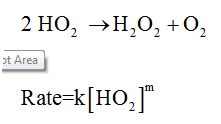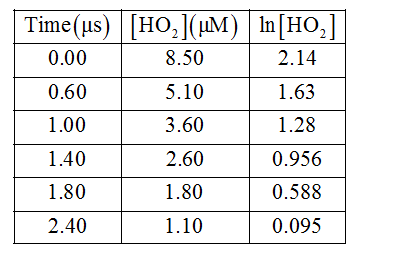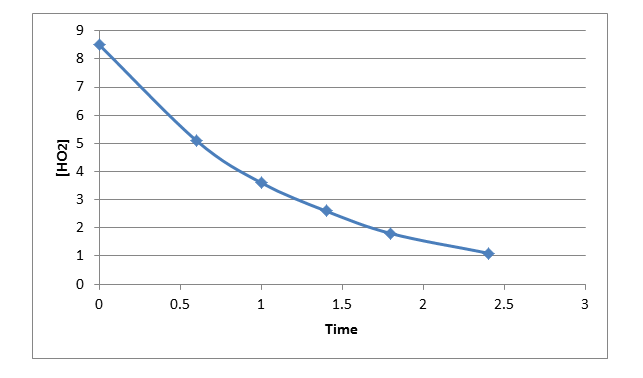# Atmospheric chemistry involves highly reactive, odd-electron molecules such as the hydroperoxyl radical HO2, which decomposes into H2O2 and O2. The following data was obtained at 298 K. Time (μμs)[HO2] (μμM)0.008.500.605.101.003.601.402.601.801.802.401.10  Determine the rate law for the reaction. Do not add multiplication symbols to your answer. Rate= Determine the value of the rate constant at 298 K.     μs-1

Question
12 views
Atmospheric chemistry involves highly reactive, odd-electron molecules such as the hydroperoxyl radical HO2, which decomposes into H2O2 and O2. The following data was obtained at 298 K.

 Time (μμs) [HO2] (μμM) 0.00 8.50 0.60 5.10 1.00 3.60 1.40 2.60 1.80 1.80 2.40 1.10

Rate=

Determine the value of the rate constant at 298 K.
μs-1

check_circle

Step 1

The balanced equation and the rate of the equation is expressed below:Step 2

Order can be determined by plotting time versus concentration and also time versus ln[conc] and it has to be checked which graph is the straight line. If the time versus concentration gives a straight line, then it will be a zero order reaction and if the time versus ln[conc] gives a straight line, then it is a first order reaction. The data is given below:Step 3

The graphs of time versus [OH2] is given below:...

### Want to see the full answer?

See Solution

#### Want to see this answer and more?

Solutions are written by subject experts who are available 24/7. Questions are typically answered within 1 hour.*

See Solution
*Response times may vary by subject and question.
Tagged in

### Chemistry